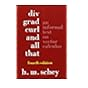Normal view

# Advanced engineering mathematics / C. Ray Wylie, Louis C. Barrett.

Material type:TextLanguage: English Publisher: New York : McGraw-Hill, c1995Edition: 6th edDescription: xxii, 1362 p. : ill. (some col.) ; 26 cmISBN: 0070722064 (acidfree paper); 9780070722064; 007113543X DDC classification: 515 LOC classification: QA401 | .W9 1995Online resources: WorldCat details
Contents:
Table of contents Ordinary differential equations of the first order -- Linear differential equations -- Complex numbers and linear algebra -- Simultaneous linear differential equations -- Numerical methods -- The descriptive theory of ordinary differential equations -- Mechanical systems and electric circuits -- Fourier series -- Fourier integrals and Fourier transforms -- The Laplace transformation -- Partial differential equations -- Bessel functions and Legendre polynomials -- Vector spaces and linear transformations -- Applications and further properties of matrices -- Vector analysis -- The calculus of variations -- Analytic functions of a complex variable -- Infinite series in the complex plane -- The theory of residues -- Conformal mapping.
Tags from this library: No tags from this library for this title.Average rating: 0.0 (0 votes)
Item type Current location Collection Call number Copy number Status Date due Barcode Item holdsText
Reserve Section
Non-fiction 515 WYA 1995 (Browse shelf) C-1 Not For Loan 12934Text
515 WYA (Browse shelf) C-2 Available 3494-12935
Total holds: 0
##### Browsing EWU Library shelves Close shelf browser
 No cover image availableNo cover image available No cover image available510 RAC College mathematical methods : 512.5 ANE Elementary linear algebra / 515 ANC Calculus / 515 WYA Advanced engineering mathematics / 515.33 EDD Differential calculus for beginners 515.35 KHD Differential equations 515.63 SCD Div, grad, curl, and all that :

Includes index.

Table of contents Ordinary differential equations of the first order --
Linear differential equations --
Complex numbers and linear algebra --
Simultaneous linear differential equations --
Numerical methods --
The descriptive theory of ordinary differential equations --
Mechanical systems and electric circuits --
Fourier series --
Fourier integrals and Fourier transforms --
The Laplace transformation --
Partial differential equations --
Bessel functions and Legendre polynomials --
Vector spaces and linear transformations --
Applications and further properties of matrices --
Vector analysis --
The calculus of variations --
Analytic functions of a complex variable --
Infinite series in the complex plane --
The theory of residues --
Conformal mapping.

Business Administration Mathematics and Physical Sciences

There are no comments for this item.

to post a comment.
Share

Library Home | Contacts | E-journals
Copyright @ 2011-2019 EWU Library
East West University